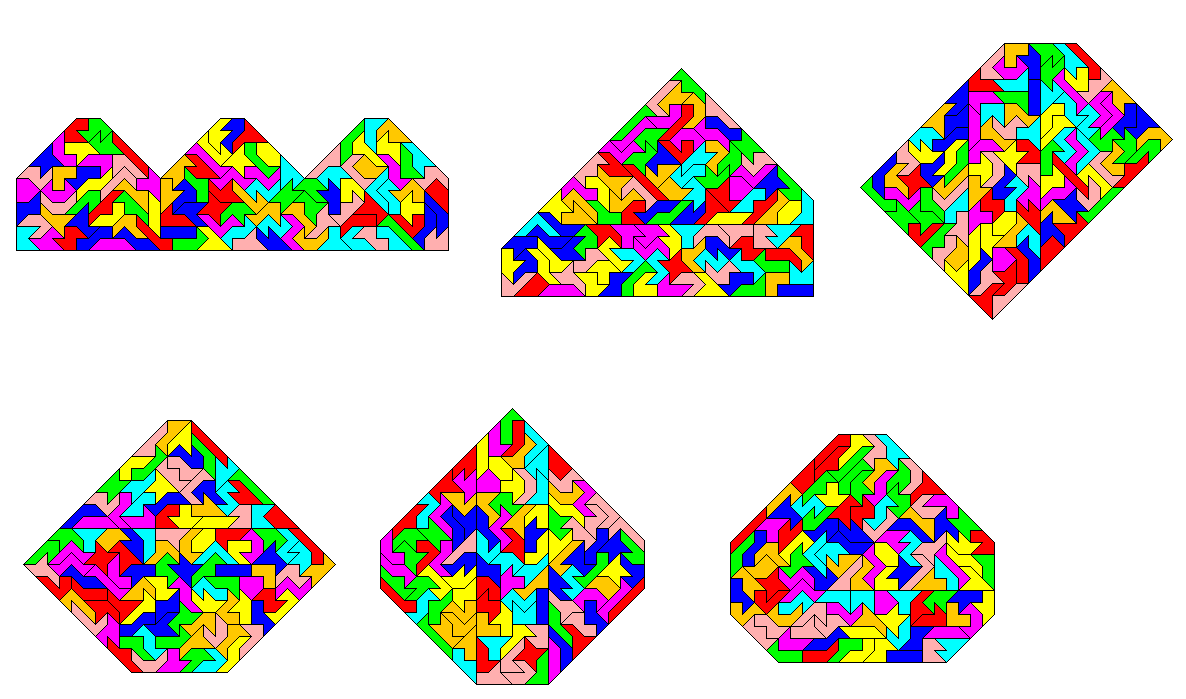## Complete Hexabolo ConstructionsAll patterns use all hexaboloes. Therefore only a limited symmetry (one axis) is possible because of the odd number of odd pieces . So I constructed a repetitive pattern (the 3 houses) and many convex patterns. A hexagon, a heptagon and a octagon with one reflection axis are shown here. You can find the only symmetric pentagon and the the only quadrangle among other extreme constructions.
The list of all convex polygons with odd numbers of parallel h-edges is very long. If you omitt all items with more than 120 h-edges or s-edges, you will get 1853 different polygons. In the range between 120 to 140 h- or s-edges there are eight polygons and I think they are impossible to build, but it isn't quite sure.
The symmetric convex polygons are listed below. The numbers are the length of the sides starting with h-units (right down), then s-units (right), then h-units (right up) and so on.

pentagons
1: 0 6 0 52 3 0 3 52 | 110s + 6h = 118,49

hexagons
1: 0 15 0 19 3 9 3 19 | 62s + 6h = 70,49
2: 0 12 3 16 3 12 0 22 | 62s + 6h = 70,49
3: 0 14 0 22 1 12 1 22 | 70s + 2h = 72,83
4: 0 13 1 21 1 13 0 23 | 70s + 2h = 72,83
5: 0 6 11 4 11 6 0 26 | 42s + 22h = 73,11
6: 9 0 12 2 12 0 9 8 | 10s + 42h = 69,40
7: 0 11 0 27 3 5 3 27 | 70s + 6h = 78,49
8: 0 8 3 24 3 8 0 30 | 70s + 6h = 78,49
9: 0 10 0 30 3 4 3 30 | 74s + 6h = 82,49
10: 0 7 3 27 3 7 0 33 | 74s + 6h = 82,49
11: 0 2 11 12 11 2 0 34 | 50s + 22h = 81,11
12: 1 0 16 2 16 0 1 32 | 34s + 34h = 82,08
13: 0 1 13 9 13 1 0 35 | 46s + 26h = 82,77
14: 0 3 7 23 7 3 0 37 | 66s + 14h = 85,80
15: 0 7 0 45 1 5 1 45 | 102s + 2h = 104,83
16: 0 6 1 44 1 6 0 46 | 102s + 2h = 104,83
17: 2 0 5 40 5 0 2 46 | 86s + 14h = 105,80
18: 0 3 3 49 3 3 0 55 | 110s + 6h = 118,49
(-- 0 2 3 60 3 2 0 66 | 130s + 6h = 138,49 --)

heptagons
1: 8 4 11 0 11 4 8 6 | 14s + 38h = 67,74
2: 2 4 13 0 13 4 2 22 | 30s + 30h = 72,43
3: 6 2 6 16 7 0 7 16 | 34s + 26h = 70,77
4: 2 6 2 28 5 0 5 28 | 62s + 14h = 81,80

octagons
1: 2 13 2 13 7 3 7 13 | 42s + 18h = 67,46
2: 2 8 7 8 7 8 2 18 | 42s + 18h = 67,46
3: 4 9 9 1 9 9 4 11 | 30s + 26h = 66,77
4: 4 6 9 4 9 6 4 14 | 30s + 26h = 66,77
5: 8 3 11 1 11 3 8 7 | 14s + 38h = 67,74
6: 2 10 2 18 5 4 5 18 | 50s + 14h = 69,80
7: 2 7 5 15 5 7 2 21 | 50s + 14h = 69,80
8: 1 11 1 21 4 5 4 21 | 58s + 10h = 72,14
9: 1 8 4 18 4 8 1 24 | 58s + 10h = 72,14
10: 6 1 7 15 7 1 6 17 | 34s + 26h = 70,77
11: 2 3 9 11 9 3 2 25 | 42s + 22h = 73,11
12: 2 2 7 20 7 2 2 30 | 54s + 18h = 79,46
13: 2 3 5 25 5 3 2 31 | 62s + 14h = 81,80

index - previous - next